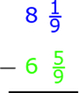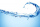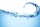# Subtract

Subtract two mixed numbers: 3 1/2 -1 3/4

x =  1.75

### Step-by-step explanation:Did you find an error or inaccuracy? Feel free to write us. Thank you!## Related math problems and questions:

• PoundsThree pounds subtract 1/3 of a pound.
• RegroupingSubtract mixed number with regrouping: 11 17/20- 6 19/20
• Fractions and mixed numerals(a) Convert the following mixed numbers to improper fractions. i. 3 5/8 ii. 7 7/6 (b) Convert the following improper fraction to a mixed number. i. 13/4 ii. 78/5 (c) Simplify these fractions to their lowest terms. i. 36/42 ii. 27/45 2. evaluate the follow
• Sum of 20Sum of the two numbers is 15 1/6. If one of the numbers is 2 2/3, find the other number.
• Divide 11Divide the product of 4 and 5/8 by 1 1/2. Write your answer as a mixed number.Add this two mixed numbers: 1 5/6 + 2 2/11=
• Mixing operations with numbersSubtract twice the number -23.6 from the difference of the numbers -130 and -40.2.
• Mixed divided with fracThere are 2 2/3 pizzas. How many people are sharing when each has 2/3 of pizza?
• Equation with mixed 2A number, X, is subtracted from 8 1/4. The result is 12 3/5. What is the value of X?
• SubtractionTest what do you know about the subtraction of two numbers: a) make a difference if the minuend 4,307,288 and subtrahend is 472008 b) minuend is 4000 more than subtrahend. What's the difference? c) the difference is 38900 less than the minuend. What is thAdd this two mixed numbers: 1 5/6 + 2 2/11=Rewrite mixed numbers, so the fractions have the same denominator: 5 1/5 - 2 2/3Benhur boiled 1 1/4 liters of water in a kettle. After 10 1/2 minutes he measured the water again. It had 3/4 liters left in the kettle. What is the amount of water that evaporates every minutes?Chin swim 9 3/4 miles a week so far this week he was swum 3 1/4 miles. How many more miles does Chin need to swim this week?The sum of 3 mixed numbers is 20 13/15. two of the numbers are 6 1/3 and 7 5/6. what is the third number?Evaluate 2x+6y when x=- 4/5 and y=1/3. Write your answer as a fraction or mixed number in simplest form.2 and 1/8th plus 1 and 1/3rd =